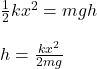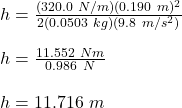## You shoot a 50.3-g pebble straight up with a catapult whose spring constant is 320.0 N/m. The catapult is initially stretched by 0.190 m. Ho

Question

You shoot a 50.3-g pebble straight up with a catapult whose spring constant is 320.0 N/m. The catapult is initially stretched by 0.190 m. How high above the starting point does the pebble fly? Ignore air resistance.

in progress 0
6 months 2021-08-22T05:40:30+00:00 1 Answers 2 views 0

## Answers ( )

The pebble reaches a height of 11.716 m above the starting point.

Explanation:

Given:

Mass of pebble (m) = 50.3 g = 0.0503 kg [1 g = 0.001 kg]

Spring constant (k) = 320.0 N/m

Elongation of catapult (x) = 0.190 m

Height of fly (h) = ?

Air resistance is ignored. So, conservation of energy holds true.

The energy stored in the catapult on stretching it is elastic potential energy. This elastic potential energy is transferred to the pebble in the form of gravitational potential energy. Therefore,

Elastic potential energy = Gravitational potential energyPlug in the given values and solve for ‘h’. This gives,Therefore, the pebble reaches a height of 11.716 m above the starting point.